CipherJan 28, 2017

CNN - How Donald Trump changed the presidency in 7 days

CNN's post - http://edition.cnn.com/2017/01/27/politics/donald-trump-first-week/index.htmlPayback time = 3+1+2+2+1+3+3 + 7+5+1+5 = 33 (Septenary)
Payback time = 7+1+7+2+1+3+11 + 2+9+4+5 = 52 (K Exception)The video in this post is 2:39 minutes long
Torah = 100+50+80+1+8 = 239 (Jewish)
Golden Gate = 7+50+20+4+5+40 + 7+1+100+5 = 239 (Jewish)

I find this very interesting
Two-three-nine = 2+5+6+2+8+9+5+5+5+9+5+5 = 66 (Reduced)
Two-three-nine = 20+23+15+20+8+18+5+5+14+9+14+5 = 156 (Ordinal)

Thirty-three = 2+8+9+9+2+7+2+8+9+5+5 = 66 (Reduced)
Thirty-three = 20+8+9+18+20+25+20+8+18+5+5 = 156 (Ordinal)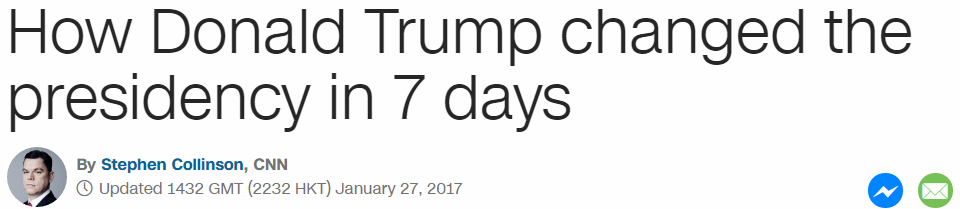How Donald Trump changed the presidency in 7 days = 197 (Reduced)Appropriate headline for the date of release - 1/27/17 = 1+27+17 = 45 - Trump is the 45th term president
seven days = 1+5+4+5+5 + 4+1+7+1 = 33 (Reduced)
This date is a match with Inauguration day as well
1/20/17 - 1+20+17 = 38 (Mr. Trump = 38)
1/27/2017 = 1+27+2+0+1+7 = 38 (Death = 38, Killing = 38), in this post we will see some numbers that go with assassinationsForget the first one hundred days = 6+15+18+7+5+20 + 20+8+5 + 6+9+18+19+20 + 15+14+5 + 8+21+14+4+18+5+4 + 4+1+25+19 = 333 (Ordinal)
Forget the first one hundred days = 6+6+9+7+5+2 + 2+8+5 + 6+9+9+1+2 + 6+5+5 + 8+3+5+4+9+5+4 + 4+1+7+1 = 144 (Reduced)
144th triangular number is 10440
Donald = 4+50+40+1+20+4 = 119 (Jewish)
Mr. Trump = 13+18 + 20+18+21+13+16 = 119 (Ordinal)
Divisors of 119 sum to 14444th prime number is 193.. Forty-four = 144 (Ordinal)
211 is 47th prime number (President = 47 Reduction)Full sentence has 271 gematria (58th prime number... Trump won the 58th presidential election and entered white house with 58 date numerology and so much more)

It doesn't matter what Trump post I start decoding, CNN has been putting the assassination codes all over him, I'm starting to wonder if I am missing something or they are truly constantly pointing to death in May...I'm gonna have to stop decoding Trump, I feel like I'm gonna be showing the same numbers until the day he dies. My prediction for May First still stands.This post is HUGE so I will just go through the subheadingsShattering the norms = 10+8+1+2+2+5+9+9+5+7 + 2+8+5 + 5+6+9+4+10 = 107 (S Exception)
Shattering the norms = 19+8+1+20+20+5+18+9+14+7 + 20+8+5 + 14+15+18+13+19 = 233 (Ordinal)
These 2 numbers coming together is extremely interesting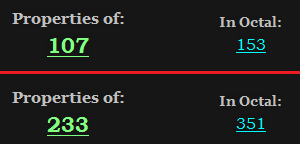28th prime number is 107 - 28 is also 2nd perfect number
233 is 13th Fibonacci number and 51st prime number
First of May = 6+9+9+1+2 + 6+6 + 4+1+7 = 51 (Reduced)
Conspiracy = 3+6+5+1+7+9+9+1+3+7 = 51 (Reduced)

Also he told ABC news that his speech was a "home run"
Home run = 8+15+13+5 + 18+21+14 = 94 (Ordinal)
Ninety-four = 40+9+40+5+100+400+6+50+200+80 = 930 (Jewish)
Divisors of 94 sum to 144
Saturn = 19+1+20+21+18+14 = 93 (Ordinal)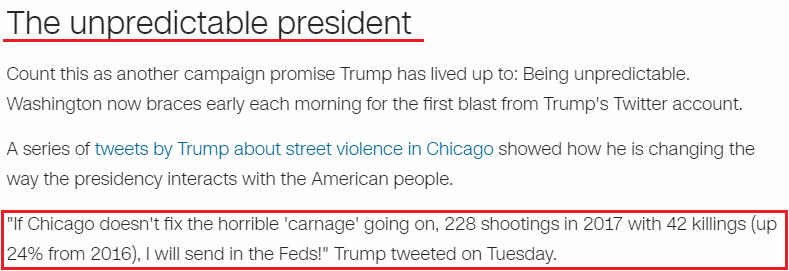The unpredictable president = 100+8+5 + 200+40+60+80+5+4+9+3+100+1+2+20+5 + 60+80+5+90+9+4+5+40+100 = 1035 (Jewish)
The unpredictable president = 20+8+5 + 21+14+16+18+5+4+9+3+20+1+2+12+5 + 16+18+5+19+9+4+5+14+20 = 273 (Ordinal)
These 2 numbers are both very interesting as well
1035 looks a lot like 135 - in my prediction he dies 135 days after start of Saturnalia in 2016

December Seventeenth = 4+5+3+5+4+2+5+9 + 10+5+4+5+5+2+5+5+5+2+8 = 93 (S Exception)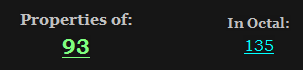135+531 = 666
Also if we measure from Trump's birthday to Saturnalia in 2017 it is 187 days much like the Homicide code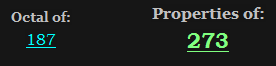The Unpredictable president = 273
Homicide code = 8+15+13+9+3+9+4+5 + 3+15+4+5 = 93 (Ordinal)
one hundred eighty seven = 6+5+5 + 8+3+5+4+9+5+4 + 5+9+7+8+2+7 + 10+5+4+5+5 = 121 (S Exception)He tweeted very interesting numbers, I don't think he even writes his own tweets... he said there was 228 shootings in 2017 with 42 killings which is 24% higher than it was in 2016
carnage = 3+1+80+40+1+7+5 = 137 (Jewish)
33rd prime number is 137

carnage = 3+1+9+5+1+7+5 = 31 (Reduced)
31st prime number is 127, note that they bring this up on 1/27
228 shootings in 2017... of course
death = 24+30+6+120+48 = 228 (Sumerian)
shootings = 90+8+50+50+100+9+40+7+90 = 444 (Jewish)
killing = 66+54+72+72+54+84+42 = 444 (Sumerian)
weapon = 138+30+6+96+90+84 = 444 (Sumerian)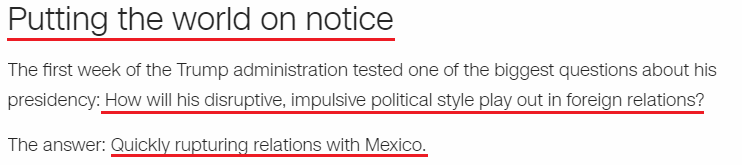Putting the world on notice = 7+3+2+2+9+5+7 + 2+8+5 + 5+6+9+3+4 + 6+5 + 5+6+2+9+3+5 = 118 (Reduced)
Death = 4+5+1+100+8 = 118 (Jewish)
A few days ago I decoded a story that came out on 1/18 full of Trump assassination codes...

Quickly rupturing relations with Mexico. = 187 (Reduced)
Imagine that... 118 followed by homicide code?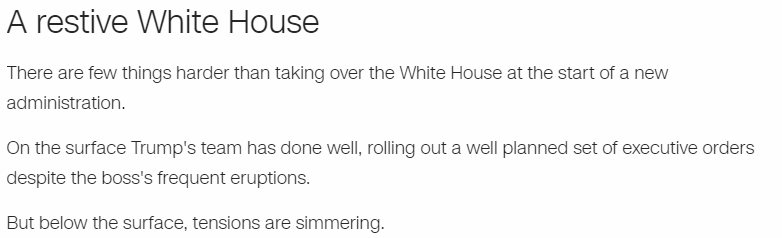A restive White House = 1 + 9+5+1+2+9+4+5 + 5+8+9+2+5 + 8+6+3+1+5 = 88 (Reduced)
Trump = 20+18+21+13+16 = 88 (Ordinal)
I won't go into more details with this post since it's one of the bigger ones I've seen

Here are the links to all Trump posts - https://nasanumerology.blogspot.rs/2017/01/artist-protests-trump-with-bloody-mural.html
https://nasanumerology.blogspot.rs/2017/01/cnn-trump-inaguration-howd-he-do.html
https://nasanumerology.blogspot.rs/2017/01/assassination-coding-for-trump-once.html
https://nasanumerology.blogspot.rs/2016/11/possible-assassination-date-for-trump.html